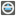• # I don't understand...

## Question related to missionFix The Generator

In the examples of the task we see: checkio([4, 2, 10]) == 1 checkio([1, 2, 3]) == 0 checkio([5, 2, 9, 6]) == 2

These examples verify only when we calculate the combinations instructed by the code:

```counter=0
for i in range(0,len(data)-2):
if data[i]+data[i+1]!=data[i+2]:
counter+=1
return counter
```

If we calculate the combinations returned using itertools.combinations the result will be increased in 4 and we 'll get assertion error...

When i try to verify the task i get an assertion error on the list [2,79,47,5,8,57,35,71,27,2] using the code above, the check result panel informs me that my result is 8 but the correct one is 101!!!

Can someone please explain to me what we have to do???